# Essbase - Formulas

Formulas calculate relationships between member in the database outline. You can :

This topic explains how TBC optimized the performance of its database by using formulas.

Formulas comprise :

• operators
• functions, as well as
• dimension names,
• member names,
• and numeric constants.

## Operators

Essbase supports the following operators:

• Mathematical operators that perform arithmetic operations
• Conditional operators that build logical conditions into calculations
• Cross-dimensional operators that point to data values of specific database member combinations

## Example

The Measures dimension uses the following formulas:

• Margin = Sales – COGS
• Total Expenses = Marketing + Payroll + Miscellaneous
• Profit = Margin – Total Expenses
• Profit % = Profit % Sales
• Margin % = Margin % Sales
• Profit per Ounce = Profit / @ATTRIBUTEVAL(@NAME(Ounces))

Essbase uses Essbase - Consolidation Operator to calculate the Margin, Total Expenses, and Profit members :

• The Margin% formula uses a % operator, which means “express Margin as a percentage of Sales”.
• The Profit% formula uses the same % operator.
• The Profit per Ounce formula uses a division operator (/) and a function (@ATTRIBUTEVAL) to calculate profitability by ounce for products sized in ounces.

In the Profit per Ounce formula, the @NAME function is also used to process the string “Ounces” for the @ATTRIBUTEVAL function.

## Documentation / Reference

• For a complete list of operators, functions, and syntax, see the Oracle Essbase Technical Reference.

Discover More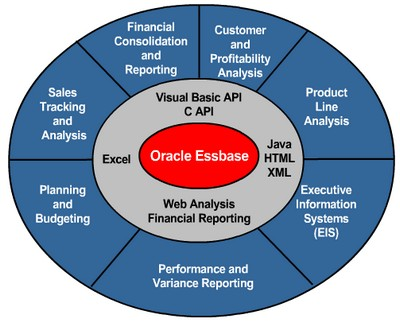Essbase - Calculations

Essbase includes powerful calculation features for demanding analytic requirements. A rich library of functions makes it easy to define advanced and sophisticated business logic and relationships. Essbase...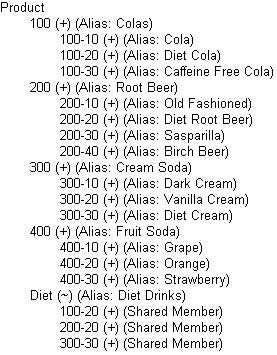Essbase - Consolidation Operator

The consolidations operator define the consolidation path and can be used in formulas. It defines how Essbase rolls up data for each member in a branch to the parent. Operator Representation Description...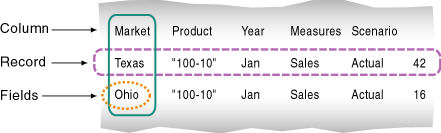Essbase - Data Source

A data source is external data that is loaded into an database with the help of one this two process : Data sources contain the information that you want to load into the Essbase database. A data...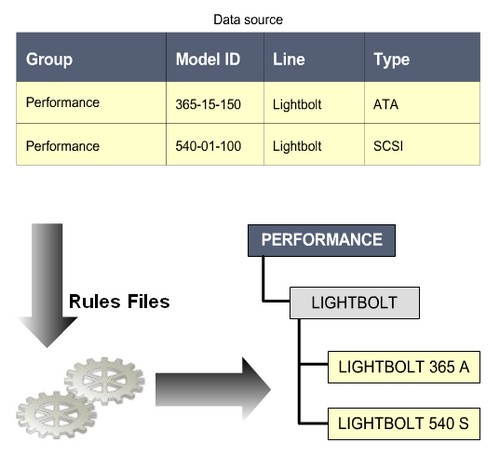Essbase - Dimension build rules files

When you create dimension with thousands of member or with many alternate hierarchy, you want to automate the process to add the member. You use Dimension build rules files to map dimension and member...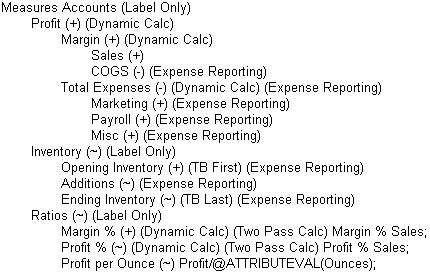Essbase - Dynamic Calculations

When you design the overall database , you may want to define a member as a Dynamic Calc member. When you a member as Dynamic Calc, Essbase calculates the of that member when you retrieve the data, instead...Essbase - MDX

in Essbase. When creating formulas for an aggregate storage database, you use MDX. When the aggregate storage option was added to Essbase, MDX was chosen over the Calc Scripting Language in order to...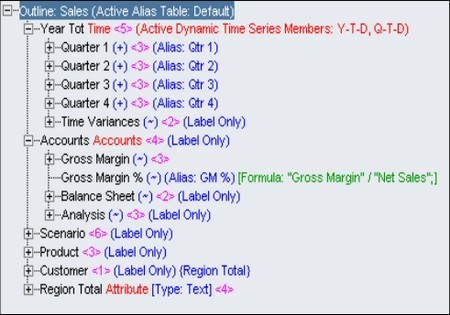Essbase - Outline (Database Outline)

An outline (also known as database outline) define the database design in a tree structure which define : dimension member consolidations formulas and calculations scripts aliases, properties,...Essbase - Outline Creation and Management using Outline Editor

This article provides an overview of creating outline using Outline Editor. For information Outline Editor, see the Oracle Essbase Administration Services Online Help. All examples in this chapter are...Essbase - Outline-based Calculations

Outline Calculations represent the simplest method of calculations. In block storage outline calculations, Essbase calculates the essbase database according to : the relationship between member in the...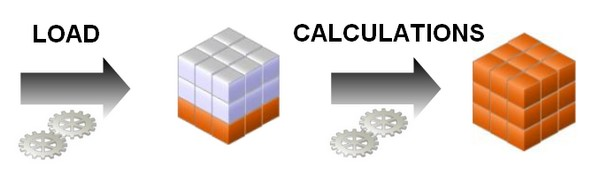Essbase - The OLAP Design Cycle (to create an optimized database)

A contains two types of values : the values that you enter or load (input data) and the values that are calculated from the input data (calculated data) Then a typical conforms to the following...